Study of mathematics online.
Study math with us and make sure that "Mathematics is easy!"

# Surface Area Formulas for geometric shapes

The surface area
is the sum of areas of all shapes that cover the surface of the geometric figure.

## Surface Area of a Cube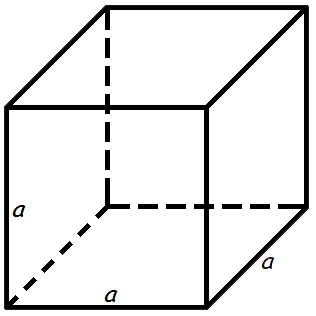The surface area of a cube is 6 times one of the sides squared.

Surface area formula of a cube:

A = 6 a2
where A - surface area of the cube,
a - the length of the side of each edge of the cube.

## Surface Area of a Rectangular Prism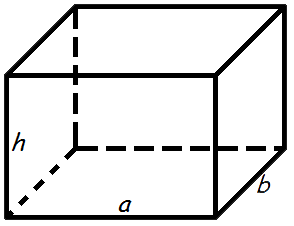The surface area of a rectangular prism is the area of the six rectangles that cover it.

Surface area formula of a rectangular prism:

A = 2(a · b + a · h + b · h)
where A - surface area of the rectangular prism,
a - length,
b - width,
h - height.

## Surface Area of a Cylinder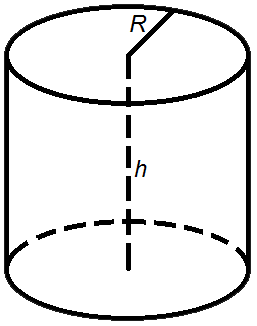Area of the cylinder side is the perimeter of top multiplied by height.

Area formula of the cylinder side:

A = 2 π R h

The surface area of a cylinder is the sum of the area of the cylinder side and two areas of top.

The formula for the surface area of a cylinder:

A = 2 π R h + 2 π R 2 = 2 π R(R + h)
where A - area,
R - the radius of the top,
h - the height of the cylinder,
π = 3.141592.

## Surface area of a Right Circular Cone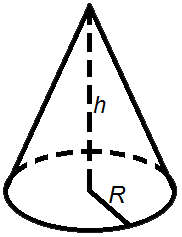The lateral surface area of a cone is slant times radius multiplied by π.

The formula for the lateral surface area of a cone:

A = π R l

The surface area of a cone is the sum of the area of the base (circular bottom) and the lateral surface area.

Surface area formula of a cone:

A = π R2 + π R l = π R (R + l)
where A - area,
R - radius of the base,
l - slant height,
π = 3.141592.

## Surface Area of a Sphere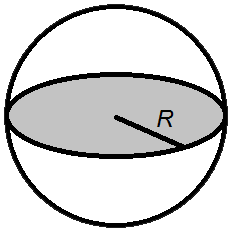Surface area formulas of a sphere:

• The surface area formulas of a sphere is 4 times radius squared multiplied by π.

A = 4 π R2
• The surface area formulas of a sphere is diameter squared multiplied by π.

A = π D2
where A - the surface area of a sphere,